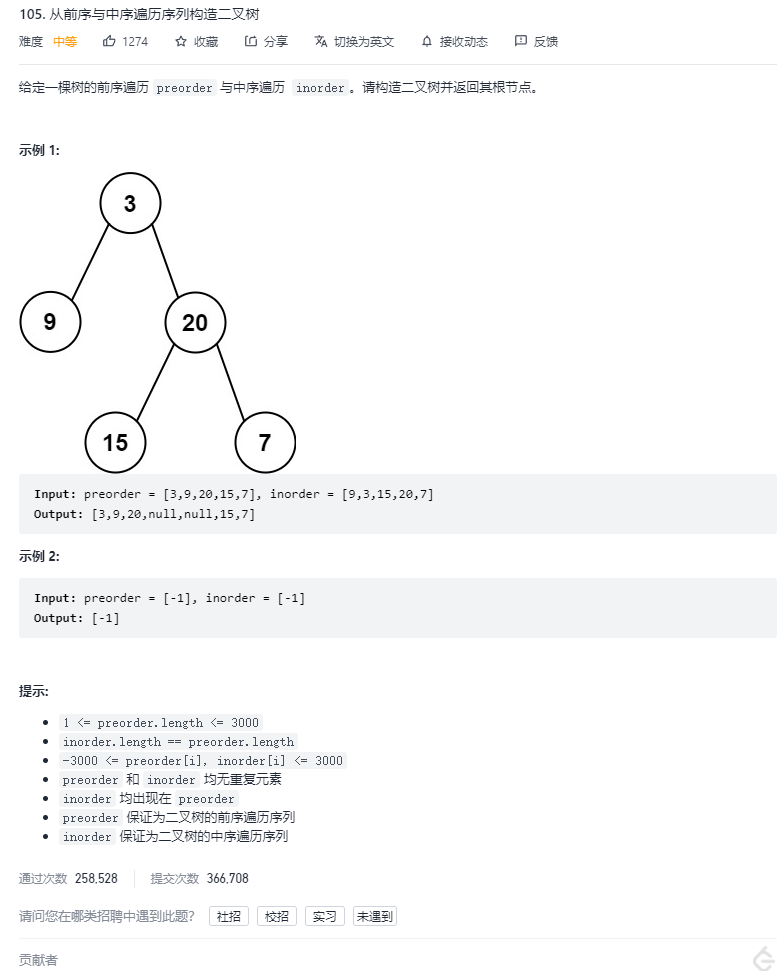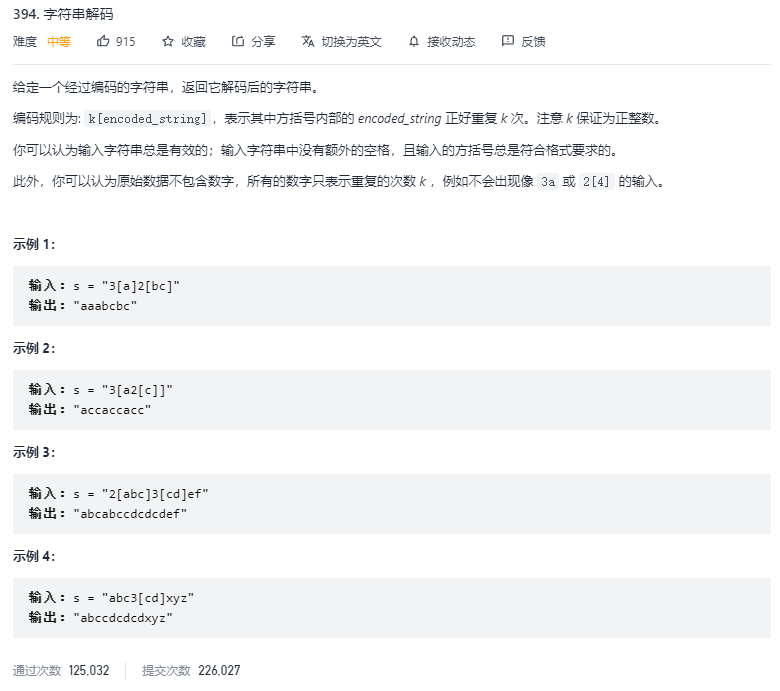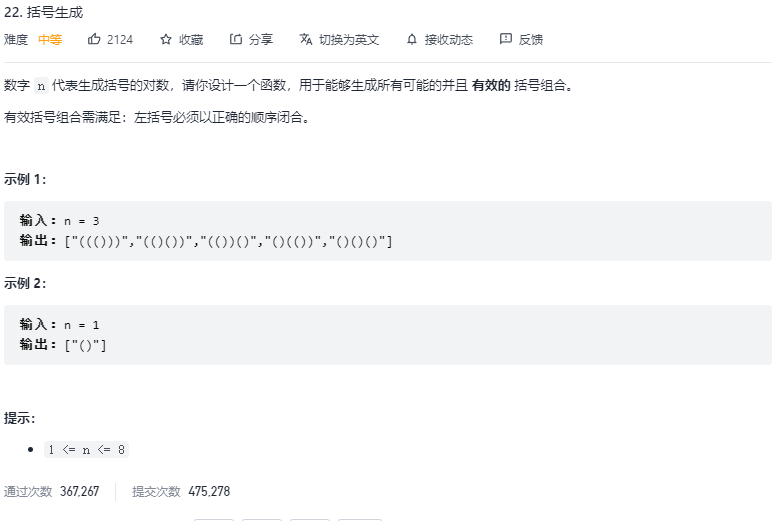## LeetCode 热题 HOT 100 ------- 22. 括号生成（回溯，dfs）394. 字符串解码(回溯) 105. 从前序与中序遍历序列构造二叉树(递归)_dasd394_slow is fast.的博客-程序员宅基地

dsaddsds``````/**
* Definition for a binary tree node.
* public class TreeNode {
*     int val;
*     TreeNode left;
*     TreeNode right;
*     TreeNode() {}
*     TreeNode(int val) { this.val = val; }
*     TreeNode(int val, TreeNode left, TreeNode right) {
*         this.val = val;
*         this.left = left;
*         this.right = right;
*     }
* }
*/

/**

*/
class Solution {

//定义一个哈希表
Map<Integer,Integer> indexMap ;

public TreeNode buildTree(int[] preorder, int[] inorder) {

//首先求得当前树一共多少个节点,也就是前序遍历数组和中序遍历数组的个数一致
int len = preorder.length;
//将中序遍历得数组放入到hashmap中
indexMap = new HashMap<Integer,Integer>();
for(int i=0 ; i<len ;i++){

indexMap.put(inorder[i],i);
}
//为了低耦合，我们用单独的方法去计算，返回的就是根节点
return myBuildTree(preorder , inorder , 0, len-1 , 0 , len-1);
}
/**
@ preorder 前序遍历的二叉树
@ inorder  右序遍历的二叉树
@ preorder_left  前序遍历的二叉树组的左边界
@ preorder_right 前序遍历的二叉树组的右边界
@ inorder_left  中序遍历的二叉树组的左边界
@ inorder_right  中序遍历的二叉树组的右边界
*/
public TreeNode myBuildTree(int[] preorder,int[] inorder,int preorder_left , int preorder_right , int inorder_left , int inorder_right){

//递归边界条件
if(preorder_left>preorder_right){

return null;
}
//前序遍历中的左边界节点就是根节点
int preorder_root = preorder_left; //根节点下标
//找对应的中序遍历数组的根节点的下标
int inorder_root = indexMap.get(preorder[preorder_root]);
//建立根节点
TreeNode root = new TreeNode(preorder[preorder_root]);
//根节点的左子树的个数就是：  -------对应的就是前序遍历数组的左边界~根节点
int size_left_subTree = inorder_root - inorder_left;
//进行递归
//先序遍历中「从 左边界+1 开始的 size_left_subtree」个元素就对应了中序遍历中「从 左边界 开始到 根节点定位-1」的元素
root.left = myBuildTree(preorder , inorder , preorder_root+1 , size_left_subTree+preorder_root , inorder_left , inorder_root-1);
//先序遍历中「从 左边界+1+左子树节点数目 开始到 右边界」的元素就对应了中序遍历中「从 根节点定位+1 到 右边界」的元素
root.right = myBuildTree(preorder , inorder ,size_left_subTree+preorder_root+1 , preorder_right , inorder_root+1 ,inorder_right);
//但是发现递归怎么结束呢？？？？找边界条件 也就是左边界<右边界
return root;
}
}

``````

dsadasd``````//解题思路：可以用辅助栈操作，或者递归
//栈操作：这个题很符合“先入后出”
//1、构建辅助栈，遍历字符串s中每个字符c
//当c为数字时，将字符转为数字multi，用于后面重复
//当c为字母时，在字母尾部添加c
//当c为‘[’时，将当前 multi 和 res 入栈，并分别置空置 0：
//当c为']'时，stack 出栈，拼接字符串 res = last_res + cur_multi * res，其中

class Solution {

public String decodeString(String s) {

//创建栈
Deque<Integer> stack_multi = new LinkedList<>();  //用于存放数字
Deque<String> stack_str = new LinkedList<>();   //用于存放字符和数字
//数字
int multi = 0;
//用于存放返回值
StringBuilder str = new StringBuilder();

for(char c : s.toCharArray()){

if( c>='0' && c<='9'){
//数字
multi = multi * 10 + Integer.parseInt(c+"");    //数字，为了避免数字是两位以上
}else if(c == '['){

multi = 0;                  //使其初始化
str = new StringBuilder();  //同样使其初始化
}else if(c == ']'){
//开始取值
StringBuilder tmp = new StringBuilder();
int cur_multi = stack_multi.removeLast(); //取']'对应的‘[’的前面的数
for(int i=0 ; i<cur_multi ; i++){

tmp.append(str);
}
//进行拼接
str = new StringBuilder(stack_str.removeLast() + tmp);
}else{
//字母
str.append(c);
}
}
return str.toString();
}
}

/**

*/
class Solution {

//递归中需要用到下标，为了方便，定义一个成员变量
private int index =0;
public String decodeString(String s) {

String str = recurve(s);
return str;
}
private String recurve(String s){

StringBuilder str = new StringBuilder();
while(index < s.length()){

char c = s.charAt(index++);
if(c>='0' && c<='9'){

int multi = c - '0';
//当遇到数字时，可能有很多，因此需要循环遍历完
while(s.charAt(index) >= '0' && s.charAt(index)<='9'){

multi = multi * 10 + s.charAt(index) - '0';
index++;
}
//然后去获得子串
String tmp = recurve(s);
for(int i=0 ; i<multi ; i++){
//将子串重复相应的次数
str.append(tmp);
}
}else if(c == '['){
//如果是[，不需要做什么

}else if(c == ']'){
//如果是]，则子串已经建立完成，退出循环
break;
}else{
//如果是普通字符，则拼接
str.append(c);
}
}
return str.toString();//返回子串
}
}
``````

dasdasdd``````//暴力搜索：先遍历出所有的括号的组合，然后判断该组合是否满足条件，满足就添加到数组 时间复杂度O(2^2n*n),空间复杂度O(n)
class Solution {

public List<String> generateParenthesis(int n) {

//返回值
List<String> str_lst = new ArrayList<>();
//每种情况
generateAllStr(new char[2*n] , 0 , str_lst);
return str_lst;
}
public void generateAllStr(char[] str_ary , int index , List<String> str_lst){

if(index == str_ary.length){

if(isVaild(str_ary)){

}
}else{

str_ary[index] = '(';
generateAllStr(str_ary,index+1,str_lst);
str_ary[index] = ')';
generateAllStr(str_ary,index+1,str_lst);
}
}
public boolean isVaild(char[] str_ary){
//判断该组合是否有效
int balance = 0;    //左括号+1，右括号-1，如果左括号小于右括号了也就是balance<0，则不能满足
for(char c : str_ary){

if(c == '('){

balance++;
}else{

balance--;
}
if(balance < 0){

return false;
}
}
return balance == 0 ? true : false;
}
}

/*
第二种方法：回溯，递归中我们每次当出现一个组合时才进行判断是否有效，
但是我们可以让其在组合其中就判断是否有效,左括号有n，右括号有n
用str代替string也行，因为每次添加string相当于创建了一个新对象，所以不需要删除
而StringBuilder这种没有创建，所以每次加完，还要删一个，不过这样写感觉就像dfs了
只不过是dfs的另一种表达
*/
class Solution {

public List<String> generateParenthesis(int n) {

List<String> str_list = new ArrayList<>();
String str = "";
recall(str_list , str , 0 , 0 , n);
return str_list;
}
public void recall(List<String> str_list , String str , int left , int right , int n){

if(str.length() == 2*n){
//进行回溯时已经保证'('<=')'
return;
}
if(left<n){
//当左括号<n的时候，我们就放左括号，然后进一步回溯 , 这里不用删除，因为每次string+括号都是新对象
recall(str_list , str +'(' , left+1 , right , n);
}
if(right<left){
//当右括号<左括号的个数时，我们就放左括号，然后进一步回溯
recall(str_list , str + ')' , left , right+1 , n);
}
}
}

/*
dfs: 和第二种差不多
有效的括号、n对，也就是说n个左括号，n个右括号，每次添加一个
但是要注意：一定要保证右括号的个数一定要少于左括号的个数，如果多了就一定不有效
*/
class Solution {

public List<String> generateParenthesis(int n) {

List<String> str_lst = new ArrayList<>();
String str = "";
dfs(str_lst , str , 0 , 0 , n);
return str_lst;
}
private void dfs(List<String> str_lst , String str , int left , int right , int n){

if(left == n && right == n){

}
// if(right > left){
//     return;
// }
if(left < n){

dfs(str_lst , str+'(' , left+1 , right , n);
}
if(right < left){

// right<n , right<left 都可以 因为上面的if(right>left) return 保证了 left<right
//所以取其1就行
dfs(str_lst , str+')' , left , right+1 , n);
}
}
}

/*
另一种方法的dfs，直接上代码吧
*/
class Solution {

public List<String> generateParenthesis(int n) {

List<String> res = new ArrayList<String>();
String str = "";
int left = n , right = n;
dfs(str , n , n , res);
return res;
}
private void dfs(String str , int l , int r , List<String> res){

//递归头
if(l == 0 && r == 0)
if(l > r)
return;
if(l>0){

dfs(str+"(" , l-1 , r , res);
}
if(r>0){

dfs(str+")" , l , r-1 , res);
}
}
}
``````

### Freemarker的FTL指令之include和if指令_ftl if-程序员宅基地

Freemarker的FTL指令之include和if指令include指令用于模板文件的嵌套_ftl if

### linux sleep cpu,Linux系统下CPU频率的调整-程序员宅基地

☆★省电or流畅★☆root@android:/sys/devices/system/cpu/cpu0/cpufreq# cat scaling_available_governorshotplug conservative ondemand userspace powersave interactive performance为了可以对几种常见的CPU频率调节模式有个基本的理解，下面简单的总...

### 【软考学习】设计模式——单例模式_单例设计模式总结-程序员宅基地

【背景】设计模式是非常重要的一块知识，每个设计模式都值得深入了解和学习。【内容】单例设计模式总结： 一、定义：保证一个类仅有一个实例，并提供一个访问它的全局观点。 二、UML结构图： 三、代码实现： using System;using System.Collections.Generic;using System.L_单例设计模式总结

### C语言中字符串的处理方式-程序员宅基地

http://www.cnblogs.com/robin-ty/archive/2010/09/03/1817294.html 交流纽带”　　　　　　　　　　　　　　　　　　　　　　　　　　　　　　--《C语言程序设计 现代方法》 　　写多了 Java 代码，对 String 类 很是喜爱，可惜经典的 C 语言没有。。。最近在做程序过程中，发现对C语言字符串的处理很模糊，一会儿..._字符串在c语言中如何处理